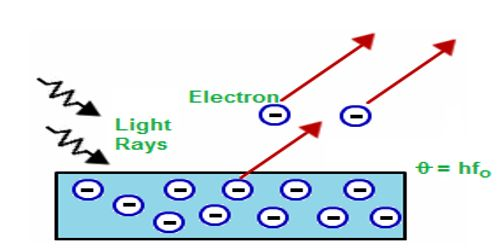# Einstein’s Photo Electric Equation

Einstein’s Photo Electric Equation

In 1905, famous scientist Einstein applied Planck’s quantum theory to explain the photoelectric effect. According to the quantum theory, and radiation is the summation of innumerable photons; that means radiation is the bundle or shower of photons. It is called photon hypothesis. If v is the frequency of the photon, then the energy of each photon = hv, here ‘h’ is the Planck’s constant. Suppose a photon of energy ‘hv’ is incident on an atom of a metallic sheet [Figure].Fig: Einstein’s Photo Electric Equation

There will be a collision between the photon and the electron and this collision will be an elastic collision. Due to this collision, an electron within the atom will absorb the whole energy of the photon and no energy will be transferred. Since the electron is bound to the nucleus. so some portion of this incident energy (W) will be used to dissociate the electron from the nucleus and the electron will be released with a velocity v along with the rest amount of energy. If the mass of the electron is m, then it’s kinetic energy = ½ mv2.

So, from the principle of conservation of energy we get,

hv = ½ mv2 + W

or, ½ mv2 = hv – W … … … (1)

Here, W = energy used to release the electron from the binding of the nucleus. When the binding energy will be minimum, then the kinetic energy of the emitted electron will have a maximum value. If this minimum binding energy is Wo and the maximum velocity of the emitted electron is vm, then equation (1) can be written as-

½ mvm2 = hv –W0 … … … (2)

This minimum binding energy is called work function. W0 is different for different materials.

Equations (1) and (2) are called the Einstein’s photoelectric equations. In the above equations, if vm = 0, then hv = W0. So, we can define work function in the following way.

Definition: The amount of energy needed to emit electron having zero velocity from the surface of a metal is called the work function of that metal.